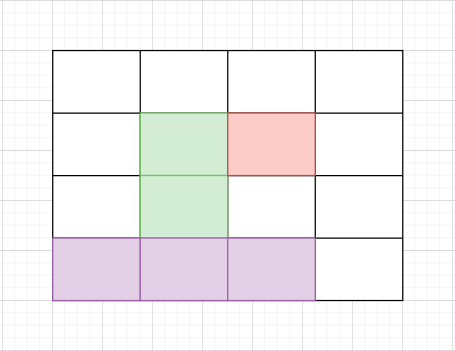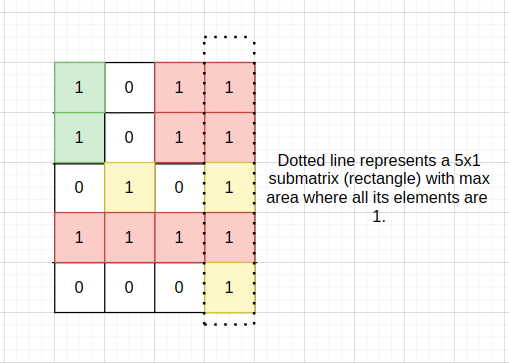7

Maximum Size Rectangle Sub-matrix With All 1's

Difficulty: HARD
Avg. time to solve
10 min
Success Rate
80%

Problem Statement

You are given an 'N' * 'M' sized binary-valued matrix 'MAT, where 'N' is the number of rows and 'M' is the number of columns. You need to return the maximum size (area) of the submatrix which consists of all 1’s i.e. the maximum area of a submatrix in which each cell has only the value ‘1’.In the above image, areas in green, red, and violet color are all submatrices of the original 4x4 matrix.

Note:

1. Binary valued matrix has only two values in each cell : 0 and 1.
2. A submatrix is a matrix formed by selecting certain rows and columns from a larger matrix.
3. The area of a matrix with 'h' rows and 'w' columns is equal to 'h' * 'w'.
Input Format:
The first line of the input contains an integer 'T' denoting the number of test cases.

The first line of each test case contains two space-separated integers 'N' and 'M', where 'N' = the number of rows in the given matrix and 'M' = the number of columns in the given matrix.

Then 'N' lines follow for each test case. Each line contains 'M' space-separated integers (either 1 or 0) denoting matrix elements.
Output Format:
For each test case print in a single line the area of maximum size submatrix of all 1’s in the given matrix on a new line.

The output of each test case will be printed in a separate line.
Note:
You do not need to print anything, it has already been taken care of. Just implement the given function.
Constraints:
1 <= 'T' <= 50
1 <= 'N', 'M' <= 100

Time Limit: 1 sec
2
2 2
1 1
1 1
5 4
1 0 1 1
1 0 1 1
0 1 0 1
1 1 1 1
0 0 0 1
4
5
Explanation For Sample Input 1:
For First Test Case: It is easy to see that whole matrix of size 2 * 2 contains '1' only hence the required area will be 4.

For Second Test Case:2
2 2
1 0
0 1
4 4
1 1 1 1
1 1 1 1
0 0 1 1
0 0 1 1
Sample Output 2:
1
8Console